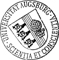## Accuracy and Stability of the Continuous-Time 3DVAR Filter for the Navier-Stokes Equation

• The 3DVAR ﬁlter is prototypical of methods used to combine observed data with a dynamical system, online, in order to improve estimation of the state of the system. Such methods are used for high dimensional data assimilation problems, such as those arising in weather forecasting. To gain understanding of filters in applications such as these, it is hence of interest to study their behaviour when applied to infinite dimensional dynamical systems. This motivates study of the problem of accuracy and stability of 3DVAR ﬁlters for the Navier-Stokes equation. We work in the limit of high frequency observations and derive continuous time filters. This leads to a stochastic partial differential equation (SPDE) for state estimation, in the form of a damped-driven Navier-Stokes equation, with mean-reversion to the signal, and spatially-correlated time-white noise. Both forward and pullback accuracy and stability results are proved for this SPDE, showing in particular that when enough lowThe 3DVAR ﬁlter is prototypical of methods used to combine observed data with a dynamical system, online, in order to improve estimation of the state of the system. Such methods are used for high dimensional data assimilation problems, such as those arising in weather forecasting. To gain understanding of filters in applications such as these, it is hence of interest to study their behaviour when applied to infinite dimensional dynamical systems. This motivates study of the problem of accuracy and stability of 3DVAR ﬁlters for the Navier-Stokes equation. We work in the limit of high frequency observations and derive continuous time filters. This leads to a stochastic partial differential equation (SPDE) for state estimation, in the form of a damped-driven Navier-Stokes equation, with mean-reversion to the signal, and spatially-correlated time-white noise. Both forward and pullback accuracy and stability results are proved for this SPDE, showing in particular that when enough low Fourier modes are observed, and when the model uncertainty is larger than the data uncertainty in these modes (variance inflation), then the filter can lock on to a small neighbourhood of the true signal, recovering from order one initial error, if the error in the observations is small. Numerical examples are given to illustrate the theory.Author: Dirk BlömkerORCiDGND, Kody Law, Andrew M. Stuart, Konstantinos C. Zygalakis urn:nbn:de:bvb:384-opus4-21310 https://opus.bibliothek.uni-augsburg.de/opus4/2131 Preprints des Instituts für Mathematik der Universität Augsburg (2012-13) Preprint English 2012 Universität Augsburg 2012/11/21 3DVAR filter; accuracy; stability Stochastische nichtlineare Differentialgleichung; Navier-Stokes-Gleichung; Filter ; Dynamisches System `Erschienen in Nonlinearity, 26, 8, S. 2193-2219, https://doi.org/10.1088/0951-7715/26/8/2193` Mathematisch-Naturwissenschaftlich-Technische Fakultät Mathematisch-Naturwissenschaftlich-Technische Fakultät / Institut für Mathematik 5 Naturwissenschaften und Mathematik / 51 Mathematik / 510 MathematikDeutsches Urheberrecht mit Print on Demand